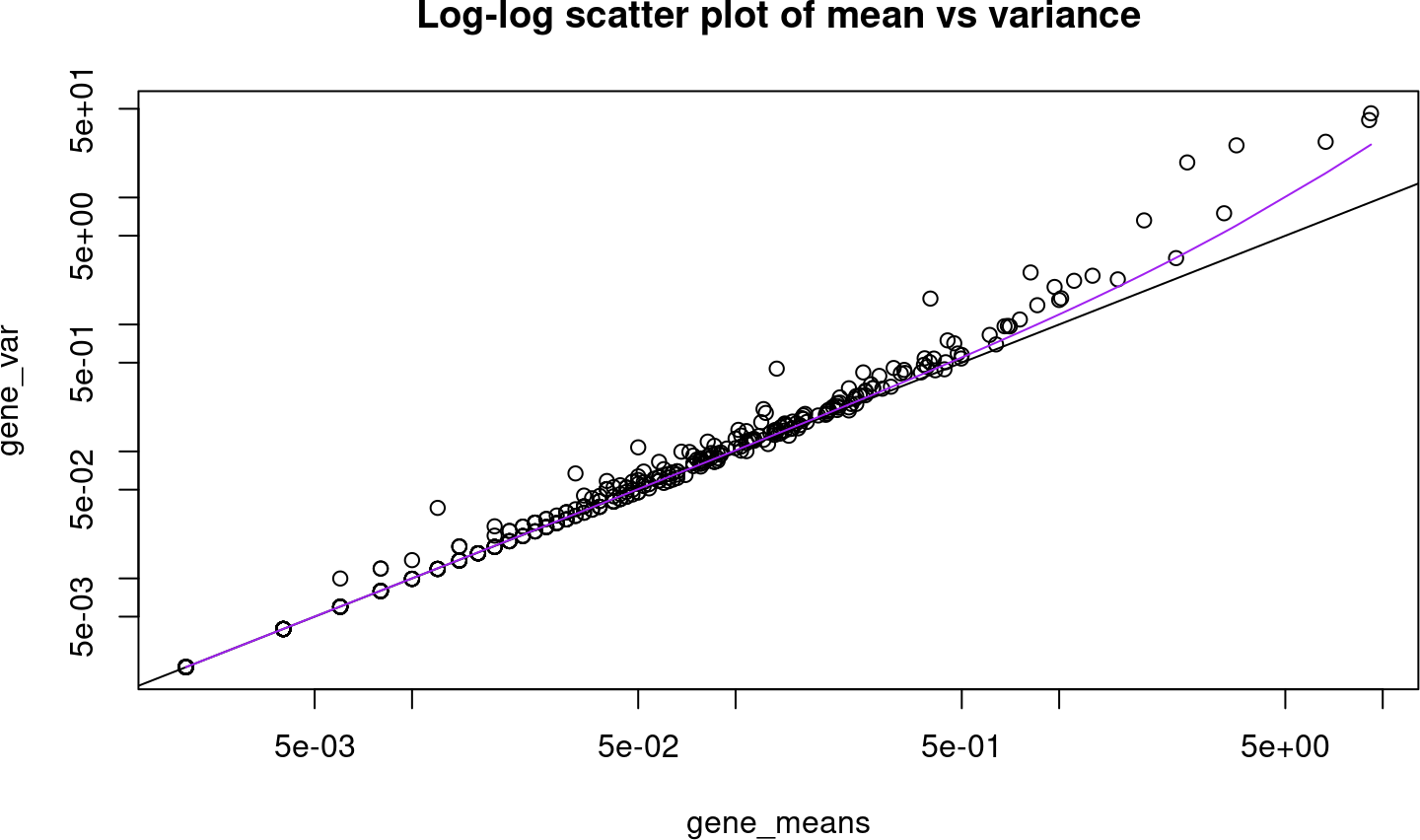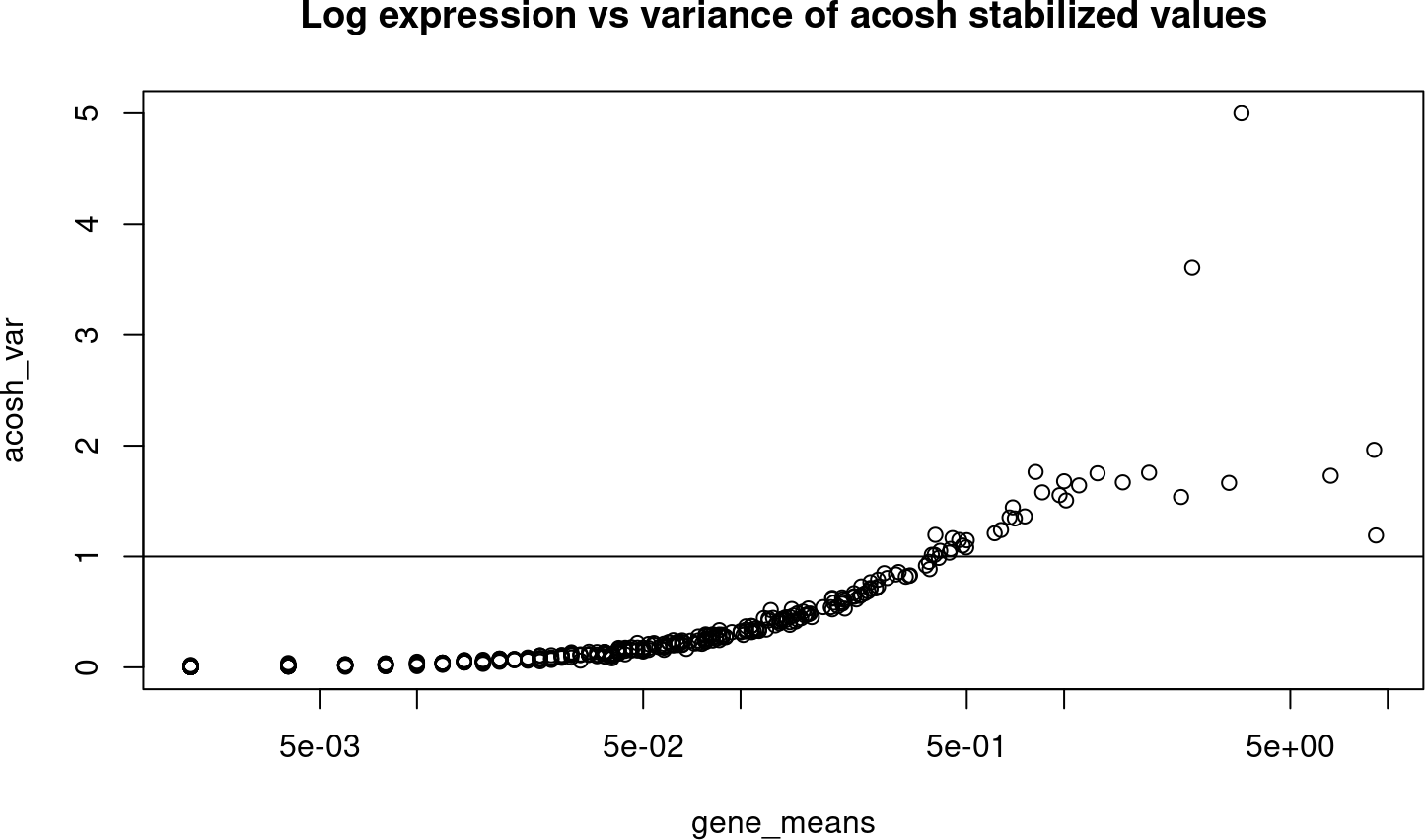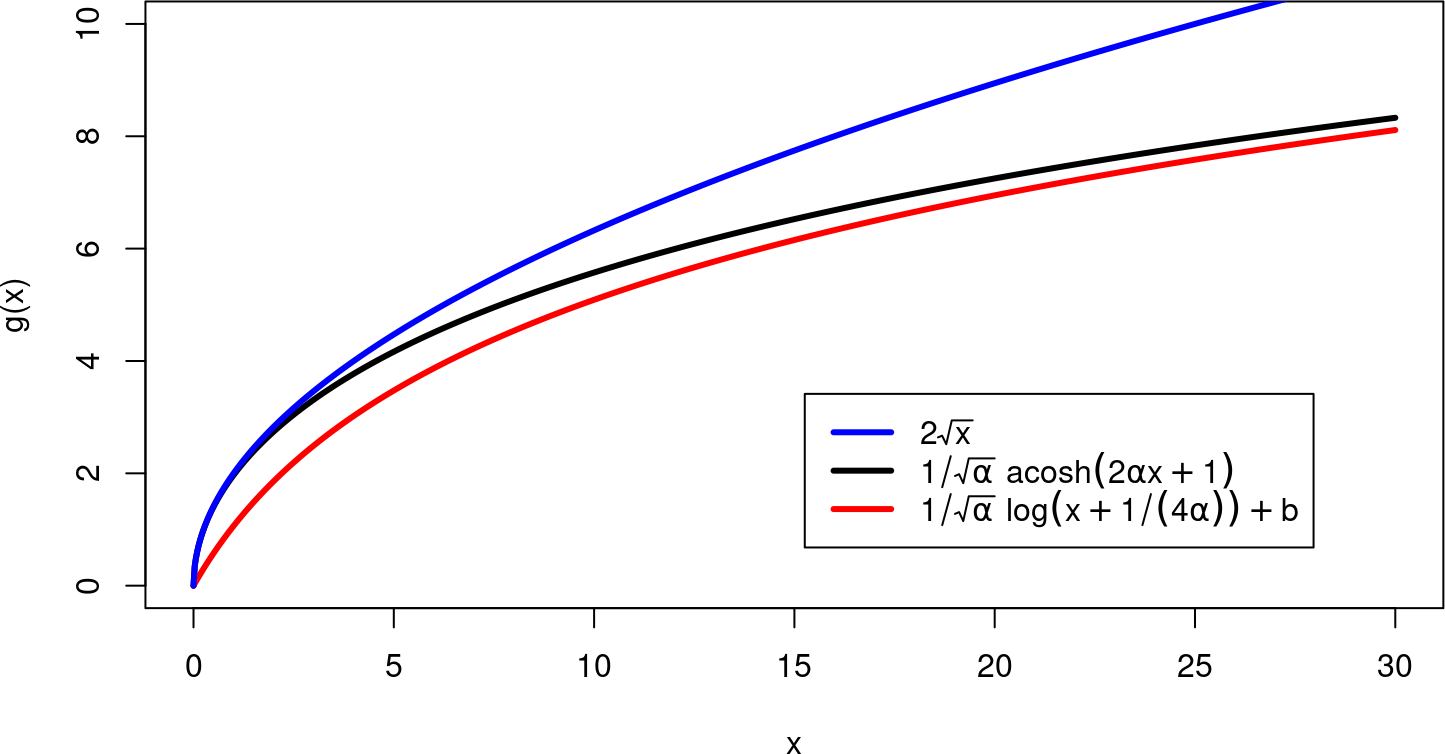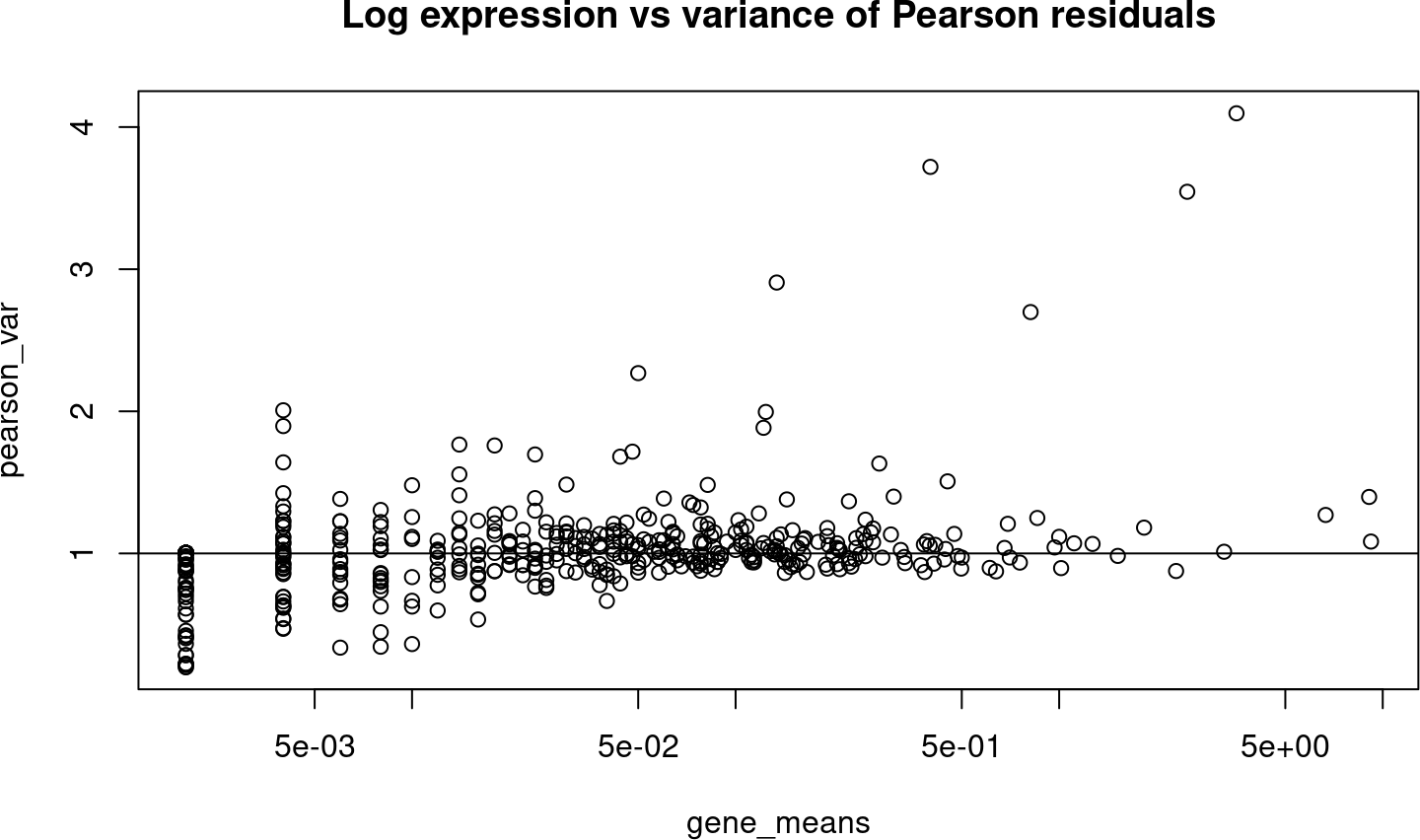# Contents

transformGamPoi provides variance stabilizing transformations to handle the heteroskedasticity of count data. For example single-cell RNA sequencing counts vary more for highly expressed genes than for lowly expressed genes. However, many classical statistical methods perform best on data with uniform variance. This package provides a set of different variance stabilizing transformations to make the subsequent application of generic statistical methods more palatable.

# 1 Installation

You can install transformGamPoi from Bioconductor after it has been accepted using the following command

if (!requireNamespace("BiocManager", quietly = TRUE))
install.packages("BiocManager")
BiocManager::install("transformGamPoi")

In the mean time or to get the latest development version, you can install transformGamPoi directly from GitHub using the devtools package

# install.packages("devtools")
devtools::install_github("const-ae/transformGamPoi")

# 2 Example

The functions in transformGamPoi take any kind of matrix-like object (e.g., matrix, dgCMatrix, DelayedArray, SummarizedExperiment, SingleCellExperiment) and return the corresponding transformed matrix objects. For sparse input the functions try to preserve sparsity. For container objects like SummarizedExperiment, transformGamPoi extracts the "counts" assay and returns an object of the same type as that "counts" assay.

We start by loading the package to make the transformation functions available in our R session:

library(transformGamPoi)

In the next step, we load some example data. Here, we use a single-cell RNA sequencing experiment of 4096 blood cells. For convenience, we subset the data to 1,000 genes and 5,00 cells

# Load the 'TENxPBMCData' as a SingleCellExperiment object
sce <- TENxPBMCData::TENxPBMCData("pbmc4k")
#> Warning: replacing previous import 'utils::findMatches' by
#> see ?TENxPBMCData and browseVignettes('TENxPBMCData') for documentation
# Subset the data to 1,000 x 500 random genes and cells
sce <- sce[sample(nrow(sce), 1000), sample(ncol(sce), 500)]

If we take a look at the stored counts (mainly zeros) stored as a sparse matrix of type DelayedMatrix. Fortunately, the precise meaning of that storage type is not important, because transformGamPoi handles this automatically.

assay(sce, "counts")[1:10, 1:5]
#> <10 x 5> sparse DelayedMatrix object of type "integer":
#>                 [,1] [,2] [,3] [,4] [,5]
#> ENSG00000167014    0    0    0    0    0
#> ENSG00000244575    0    0    0    0    0
#> ENSG00000118495    0    0    0    0    0
#> ENSG00000140479    0    0    0    0    0
#> ENSG00000236641    0    0    0    0    0
#> ENSG00000131174    0    1    2    0    0
#> ENSG00000250905    0    0    0    0    0
#> ENSG00000162040    0    0    0    0    0
#> ENSG00000140557    0    0    0    0    0
#> ENSG00000273523    0    0    0    0    0

To see what we mean by heteroskedasticity, let us compare the mean and variance for each gene across cells. We will use the MatrixGenerics package to calculate the row means and row variances. You might be familiar with the matrixStats package; MatrixGenerics provides the same set of functions but depending on the type of the matrix automatically dispatches the call to either matrixStats, DelayedMatrixStats, or sparseMatrixStats.

library(MatrixGenerics)
# Exclude genes where all counts are zero
sce <- sce[rowMeans2(counts(sce)) > 0, ]
#> Registered S3 method overwritten by 'SparseArray':
#>   method           from
#>   rowsum.dgCMatrix DelayedArray
gene_means <- rowMeans2(counts(sce))
gene_var <- rowVars(counts(sce))
plot(gene_means, gene_var, log = "xy", main = "Log-log scatter plot of mean vs variance")
abline(a = 0, b = 1)
sorted_means <- sort(gene_means)
lines(sorted_means, sorted_means + 0.2 * sorted_means^2, col = "purple")The purple line shows a quadratic mean-variance relation ($$\text{Var} = \mu + 0.2 \mu^2$$) typical for data that is Gamma-Poisson distributed. For example a gene with a mean expression of 5 the corresponding variance is 10, whereas for a gene with a mean expression of 500 the variance ~50,000. Here we used an overdispersion of $$\alpha = 0.2$$, transformGamPoi provides options to either fit $$\alpha$$ on the data or fix it to a user-defined value.

transformGamPoi implements two approaches for variance stabilization: (1) based on the delta method, (2) based on model residuals.

## 2.1 Delta method-based variance stabilizing transformations

The delta method relates the standard deviation of a transformed random variable $$g(X_i)$$ to the standard deviation of the original random variable $$X_i$$. This can be used to find a function such that $$g(X_i) = \text{const}$$. For a quadratic mean variance relation this function is $g(x) = \frac{1}{\sqrt{\alpha}} \text{acosh}(2 \alpha x + 1).$

We can apply this transformation to the counts:

assay(sce, "acosh") <- acosh_transform(assay(sce, "counts"))
# Equivalent to 'assay(sce, "acosh") <- acosh_transform(sce)'

We plot the variance of the acosh transformed counts and find that for $$\mu < 0.5$$ the variance still increases for higher average gene expression. However, for larger expression values the variance for a gene is approximately independent of the corresponding average gene expression (note that the y-axis is not log transformed anymore!).

acosh_var <- rowVars(assay(sce, "acosh"))
plot(gene_means, acosh_var, log = "x", main = "Log expression vs variance of acosh stabilized values")
abline(h = 1)The most popular transformation for single cell data is $$g(x) = \log(x + c)$$ with pseudo-count $$c=1$$. It turns out that this transformation is closely related to the acosh transformation. When we choose $$c = 1/(4\alpha)$$ the two converge rapidly, only for small values the acosh is closer to $$g(x) = 2\sqrt{x}$$:

x <- seq(0, 30, length.out = 1000)
y_acosh <- acosh_transform(x, overdispersion = 0.1)
y_shiftLog <- shifted_log_transform(x, pseudo_count = 1/(4 * 0.1))
y_sqrt <- 2 * sqrt(x) # Identical to acosh_transform(x, overdispersion = 0)

The plot looks as follows:

plot(x, y_acosh, type = "l", col = "black", lwd = 3, ylab = "g(x)", ylim = c(0, 10))
lines(x, y_shiftLog, col = "red", lwd = 3)
lines(x, y_sqrt, col = "blue", lwd = 3)
legend("bottomright", legend = c(expression(2*sqrt(x)),
expression(1/sqrt(alpha)~acosh(2*alpha*x+1)),
expression(1/sqrt(alpha)~log(x+1/(4*alpha))+b)),
col = c("blue", "black", "red"), lty = 1, inset = 0.1, lwd = 3)The offset $$b$$ for the shifted logarithm has no influence on the variance stabilization. We choose $$b$$ such that sparsity of the input is retained (i.e., $$g(0) = 0$$).

## 2.2 Model residuals-based variance stabilizing transformations

An alternative approach for variance stabilization was suggested by Hafemeister and Satija (2019). They used the Pearson residuals from a Gamma-Poisson generalized linear model fit as the variance stabilized values. The advantage of this approach is that the variance is also stabilized for lowly expressed genes unlike the delta method-based transformations:

assay(sce, "pearson") <- residual_transform(sce, "pearson", clipping = TRUE, on_disk = FALSE)
pearson_var <- rowVars(assay(sce, "pearson"))
plot(gene_means, pearson_var, log = "x", main = "Log expression vs variance of Pearson residuals")
abline(h = 1)Pearson residuals are by definition a linear transformation. This means that for genes with strong expression differences across subgroups they cannot achieve variance stabilization. As an alternative, transformGamPoi provides randomized quantile residuals which are non-linear and exploit randomization to work around the discrete nature of counts:

assay(sce, "rand_quantile") <- residual_transform(sce, "randomized_quantile", on_disk = FALSE)
rand_quant_var <- rowVars(assay(sce, "rand_quantile"))
plot(gene_means, rand_quant_var, log = "x", main = "Log expression vs variance of Randomized Quantile residuals")
abline(h = 1)